# Round a Float to 1, 2 or 3 Decimal places in PythonLast updated: Jan 27, 2023
7 min## #Round a float to 1, 2 or 3 decimal places in Python

Use the `round()` function to round a float to 1, 2 or 3 decimal places, e.g. `result = round(6.36789, 2)`.

The `round()` function will round the floating-point number to the specified number of decimal places and will return the result.

main.py
```Copied!```my_float = 6.36789

# ✅ round a float to 1, 2 or 3 decimal places (round())

result = round(my_float, 1)
print(result)  # 👉️ 6.4

result = round(my_float, 2)
print(result)  # 👉️ 6.37

result = round(my_float, 3)
print(result)  # 👉️ 6.368
``````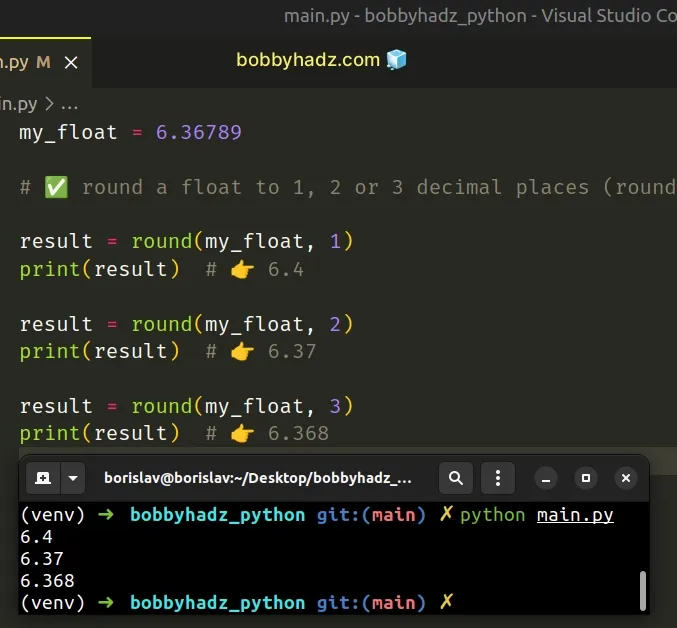The example uses the `round()` function to round a floating-point number to the specified number of decimal places.

main.py
```Copied!```my_float = 6.36789

result = round(my_float, 3)
print(result)  # 👉️ 6.368
``````

The round function takes the following 2 parameters:

NameDescription
`number`the number to round to `ndigits` precision after the decimal
`ndigits`the number of digits after the decimal, the number should have after the operation (optional)

The `round` function returns the number rounded to `ndigits` precision after the decimal point.

If you need to call the `round()` function on a list of floats, use a list comprehension.

main.py
```Copied!```list_of_floats = [1.42342365, 3.438834, 5.5843854]

result = [round(num, 2) for num in list_of_floats]

print(result) # 👉️ [1.42, 3.44, 5.58]
``````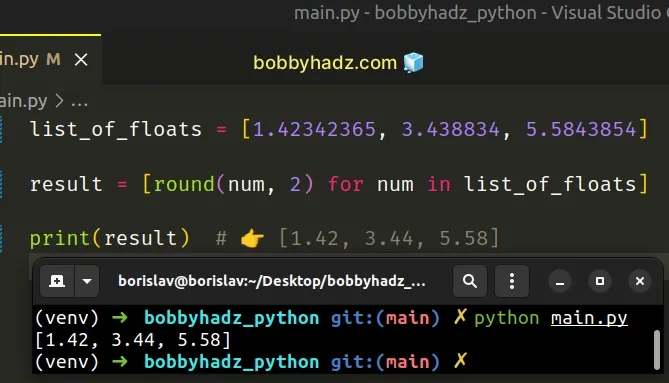We used a list comprehension to iterate over the list of floating-point numbers and passed each number to the `round()` function.

## #Round a float to 1, 2 or 3 decimal places using f-string

Alternatively, you can use a formatted string literal.

main.py
```Copied!```my_float = 6.36789

result = f'{my_float:.1f}'
print(result)  # 👉️ '6.4'

result = f'{my_float:.2f}'
print(result)  # 👉️ '6.37'

result = f'{my_float:.3f}'
print(result)  # 👉️ '6.368'

number_of_decimals = 3

result = f'{my_float:.{number_of_decimals}f}'
print(result)  # 👉️ '6.368'
``````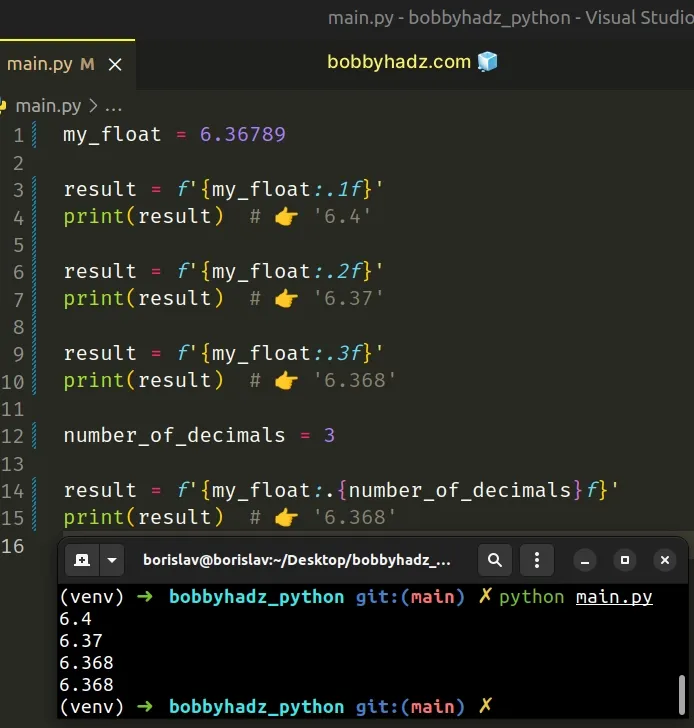Formatted string literals (f-strings) let us include expressions inside of a string by prefixing the string with `f`.

Make sure to wrap expressions in curly braces - `{expression}`.

Formatted string literals also enable us to use the format-specific mini-language in expression blocks.

main.py
```Copied!```my_float = 6.36789

# 👇️ Rounded to 1 decimals: 6.4
print(f'Rounded to 2 decimals: {my_float:.1f}')

# 👇️ Rounded to 2 decimals: 6.37
print(f'Rounded to 2 decimals: {my_float:.2f}')

# 👇️ Rounded to 3 decimals: 6.368
print(f'Rounded to 3 decimals: {my_float:.3f}')
``````

The digit after the period is the number of decimal places the float should have.

If you have the number of decimal places stored in a variable, wrap it in curly braces in the f-string.

main.py
```Copied!```my_float = 6.36789

number_of_decimals = 3

result = f'{my_float:.{number_of_decimals}f}'
print(result)  # 👉️ '6.368'
``````

If you need to round a list of floating-point numbers to N decimal places, use a list comprehension.

main.py
```Copied!```my_float = 6.36789

list_of_floats = [2.298438438, 4.5848548, 8.8347347]

result = [f'{item:.3f}' for item in list_of_floats]
print(result)  # 👉️ ['2.298', '4.585', '8.835']
``````

We used a list comprehension to iterate over the list of floating-point numbers.

List comprehensions are used to perform some operation for every element or select a subset of elements that meet a condition.

On each iteration, we use a formatted string literal to round the current float to 3 decimal places and return the result.

## #Round down float to 1, 2 or 3 decimals in Python

Rounding a float down to N decimals is a three-step process:

1. Call the `math.floor()` method, passing it the number multiplied by `100`.
2. Divide the result by `100`.
3. The result of the calculation will be the float number rounded down to 2 decimals.
main.py
```Copied!```import math

def round_down_float_to_2_decimals(num):
return math.floor(num * 100) / 100

print(round_down_float_to_2_decimals(4.6789))  # 👉️ 4.67
``````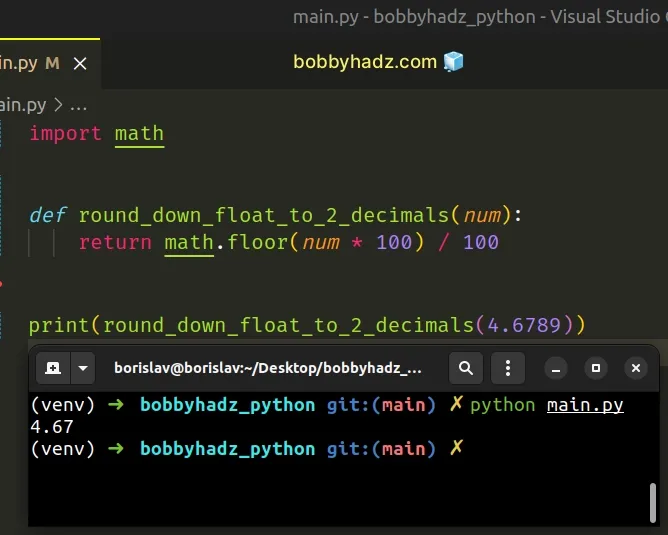The same approach can be used to round down a float to 3 decimal places.

main.py
```Copied!```import math

def round_down_float_to_3_decimals(num):
return math.floor(num * 1000) / 1000

print(round_down_float_to_3_decimals(4.6789))  # 👉️ 4.678
``````

The same approach can be used to round down a float to 1 decimal place.

main.py
```Copied!```import math

def round_down_float_to_1_decimal(num):
return math.floor(num * 10) / 10

print(round_down_float_to_1_decimal(2.6789))  # 👉️ 2.6
``````

The math.floor method returns the largest integer less than or equal to the provided number.

main.py
```Copied!```import math

print(math.floor(15.999))  # 👉️ 15
print(math.floor(15.001))  # 👉️ 15
``````
If the passed-in number has a fractional part, the `math.floor` method rounds the number down.

Here is a step-by-step example of rounding down a float to 2 decimal places.

main.py
```Copied!```import math

print(4.4567 * 100)  # 👉️ 445.66999999999996
print(4.1234 * 100)  # 👉️ 412.34000000000003

print(math.floor(4.4567 * 100))  # 👉️ 445
print(math.floor(4.1234 * 100))  # 👉️ 412

print(math.floor(4.4567 * 100) / 100)  # 👉️ 4.45
print(math.floor(4.1234 * 100) / 100)  # 👉️ 4.12
``````
We first multiply the number by `100` and then divide by `100` to shift 2 decimal places to the left and right, so that `math.floor()` works on the hundreds.

This is a two-step process:

1. Multiply the number by `100` and round the result down to the nearest integer.
2. Divide the result by `100` to round down the float to 2 decimal places.

## #Round up float to 1, 2 or 3 decimals in Python

Rounding up a float to N decimals is a three-step process:

1. Call the `math.ceil()` method, passing it the number multiplied by `100`.
2. Divide the result by `100`.
3. The result of the calculation will be the float number rounded up to 2 decimals.
main.py
```Copied!```import math

def round_up_float_to_2_decimals(num):
return math.ceil(num * 100) / 100

print(round_up_float_to_2_decimals(4.6123))  # 👉️ 4.62
``````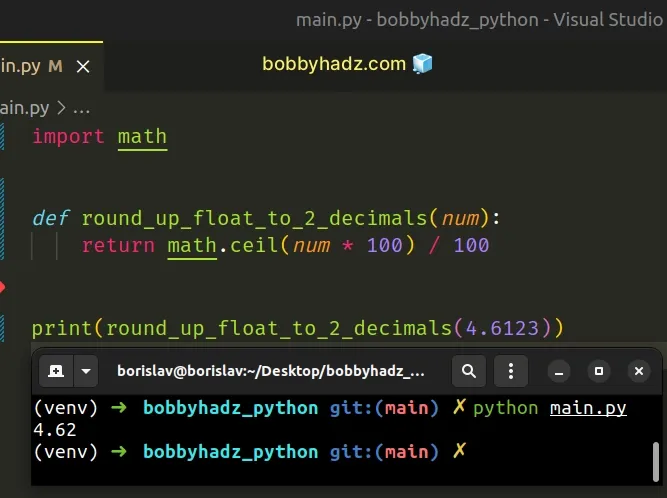The same approach can be used to round up a float to 3 decimal places.

main.py
```Copied!```import math

def round_up_float_to_3_decimals(num):
return math.ceil(num * 1000) / 1000

print(round_up_float_to_3_decimals(4.6123))  # 👉️ 4.62
``````

The same approach can be used to round up a float to 1 decimal place.

main.py
```Copied!```import math

def round_up_float_to_1_decimal(num):
return math.ceil(num * 10) / 10

print(round_up_float_to_1_decimal(2.6123))  # 👉️ 2.7
``````

The math.ceil method returns the smallest integer greater than or equal to the provided number.

main.py
```Copied!```import math

print(math.ceil(76.001))  # 👉️ 77
print(math.ceil(76.999))  # 👉️ 77
``````

If the passed-in number has a fractional part, the `math.ceil` method rounds the number up.

Here is a step-by-step example of rounding up a float to 2 decimal places.

main.py
```Copied!```import math

print(4.4567 * 100)  # 👉️ 445.66999999999996
print(4.1234 * 100)  # 👉️ 412.34000000000003

print(math.ceil(4.4567 * 100))  # 👉️ 446
print(math.ceil(4.1234 * 100))  # 👉️ 413

print(math.ceil(4.4567 * 100) / 100)  # 👉️ 4.46
print(math.ceil(4.1234 * 100) / 100)  # 👉️ 4.13
``````
We first multiply the number by `100` and then divide by `100` to shift 2 decimal places to the left and right, so that `math.ceil()` works on the hundreds.

This is a two-step process:

1. Multiply the number by `100` and round the result up to the nearest integer.
2. Divide the result by `100` to round up the float to 2 decimal places.

## #Round a list of floats to 2 decimal places in Python

To round a list of floats to 2 decimal places:

1. Use a list comprehension to iterate over the list.
2. Use the `round()` function to round each float to 2 decimal places.
3. The new list will contain floating-point numbers rounded to 2 decimals.
main.py
```Copied!```list_of_floats = [2.4834843583, 4.28384291, 8.47989238]

# ✅ Round list of floats to 2 decimal places (round())
result = [round(item, 2) for item in list_of_floats]
print(result)  # 👉️ [2.48, 4.28, 8.48]

# ------------------------------------------

# ✅ Format list of floats to 2 decimal places (f-string)

result = [f'{item:.2f}' for item in list_of_floats]
print(result)  # 👉️ ['2.48', '4.28', '8.48']

# ------------------------------------------

# ✅ Round list of floats to 2 decimal places (numpy.around())

import numpy as np

result = list(np.around(list_of_floats, 2))
print(result)  # 👉️ [2.48, 4.28, 8.48]
``````

We used a list comprehension to iterate over the list of floating-point numbers.

main.py
```Copied!```list_of_floats = [2.4834843583, 4.28384291, 8.47989238]

result = [round(item, 2) for item in list_of_floats]
print(result)  # 👉️ [2.48, 4.28, 8.48]
``````
List comprehensions are used to perform some operation for every element or select a subset of elements that meet a condition.

On each iteration, we use the `round()` function to round the current float to 2 decimal places.

The round function takes the following 2 parameters:

NameDescription
`number`the number to round to `ndigits` precision after the decimal
`ndigits`the number of digits after the decimal, the number should have after the operation (optional)

The `round` function returns the number rounded to `ndigits` precision after the decimal point.

Alternatively, you can use a formatted string literal.

## #Round a list of floats to 2 decimal places using f-string

This is a three-step process:

1. Use a list comprehension to iterate over the list.
2. Use a formatted-string literal to round each float to 2 decimal places.
3. The new list will contain floating-point numbers rounded to 2 decimals.
main.py
```Copied!```list_of_floats = [2.4834843583, 4.28384291, 8.47989238]

result = [f'{item:.2f}' for item in list_of_floats]
print(result)  # 👉️ ['2.48', '4.28', '8.48']
``````
Formatted string literals (f-strings) let us include expressions inside of a string by prefixing the string with `f`.

Make sure to wrap expressions in curly braces - `{expression}`.

Formatted string literals also enable us to use the format-specific mini-language in expression blocks.

main.py
```Copied!```my_float = 2.4834843583

print(f'{my_float:.2f}')  # 👉️ 2.48
print(f'{my_float:.3f}')  # 👉️ 2.483
``````

The digit after the period is the number of decimal places the float should have.

If you have the number of decimal places stored in a variable, wrap it in curly braces in the f-string.

main.py
```Copied!```list_of_floats = [2.4834843583, 4.28384291, 8.47989238]
number_of_decimals = 2

result = [f'{item:.{number_of_decimals}f}' for item in list_of_floats]
print(result)  # 👉️ ['2.48', '4.28', '8.48']
``````

If you use NumPy, you can use the `numpy.around()` method.

## #Round a list of floats to 2 decimal places using numpy.around()

This is a two-step process:

1. Use the `numpy.around()` method to round each float in the list to 2 decimal places.
2. Use the `list()` class to convert the NumPy array to a list.
main.py
```Copied!```import numpy as np

list_of_floats = [2.4834843583, 4.28384291, 8.47989238]

result = list(np.around(list_of_floats, 2))
print(result)  # 👉️ [2.48, 4.28, 8.48]
``````

Make sure you have the NumPy module installed to be able to run the code sample:

shell
```Copied!```pip install numpy

pip3 install numpy
``````

The numpy.around method takes an array-like object and rounds its items to the given number of decimals.

The `decimals` argument is `0` by default.

The last step is to use the `list()` class to convert the NumPy array to a list.

The list class takes an iterable and returns a list object.

I've also written an article on how to round a number to the nearest 5, 10, 100, 1000.